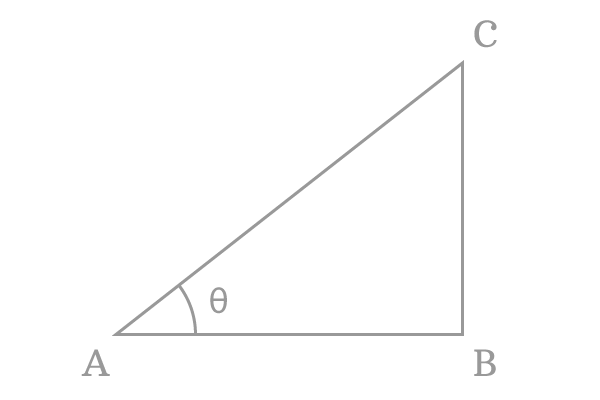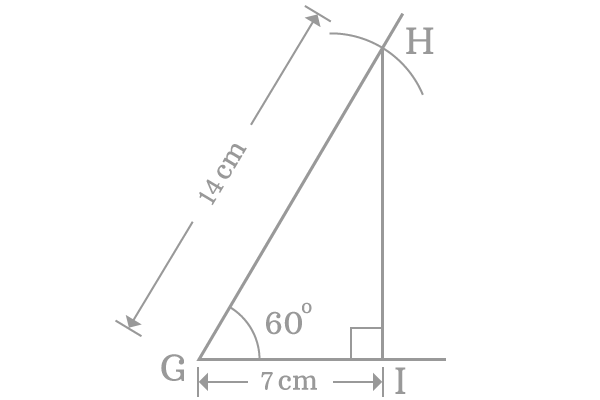# Inverse cosine function

## Definition

The inverse of the cosine function is called the inverse cosine function.

The cosine function represents a value of the ratio of length of the adjacent side to length of the hypotenuse at the associated angle of the triangle, whereas the inverse cosine function represents an angle of the triangle for a value of the ratio of the length of the adjacent side to length of the hypotenuse of the same right angled triangle.

### Function

The inverse cosine is written in short form as $\large \arccos$ or $\large \cos^{-1}$ in mathematics.

As per the fundamental definition of the inverse cosine function, it is written in mathematical form in the following ways.

$\large \cos^{-1} \normalsize \Bigg[\dfrac{Length \, of \, Adjacent \, side}{Length \, of \, Hypotenuse}\Bigg]$ $\,=\,$ $Angle \, of \, the \, Triangle$

It is also written in the following form.

$\large \arccos \normalsize \Bigg[\dfrac{Length \, of \, Adjacent \, side}{Length \, of \, Hypotenuse}\Bigg]$ $\,=\,$ $Angle \, of \, the \, Triangle$For example, $\Delta CAB$ is a right angled triangle and the angle of the triangle is theta ($\theta$). $\overline{AB}$ and $\overline{AC}$ are adjacent side and hypotenuse and their lengths are represented by $AB$ and $AC$ respectively.

Write the arc cosine function in terms of sides of the triangle $CAB$ to express it in mathematical form.

$\large \cos^{-1} \normalsize \Big(\dfrac{AB}{AC}\Big) \,=\, \theta \,\,\,$ or $\,\,\, \large \arccos \normalsize \Big(\dfrac{AB}{AC}\Big) \,=\, \theta$

Consider $x$ as the quotient of the length of the adjacent side by the length of the hypotenuse.

Then, $\large \cos^{-1} x \,=\, \theta \,\,\,$ or $\,\,\, \large \arccos x \,=\, \theta$

Thus, an inverse cosine function is denoted as $\cos^{-1} x$ or $\arccos x$ in mathematical form.

#### Example

The functionality of the inverse cosine function can be studied from an example.1. Take ruler and draw a horizontal line and assume the name of left side point is $G$.
2. Take protractor and draw a line with $60^\circ$ angle from point $G$.
3. After that, take compass and set its distance to $14$ cm with the help of a ruler and then draw an arc on $60^\circ$ angle line from point $G$. Call the intersecting point as point $H$.
4. Draw a line from point $H$ to horizontal line but it should intersect the horizontal line perpendicularly at point $I$.

Thus a right angled triangle $HGI$ is constructed geometrically and now measure the length of the adjacent side ($GI$). It should be $7 \, cm$ exactly. The angle of the triangle is $60^\circ$.

Now, express the inverse cosine function in terms of ratio of sides and the respective angle of the triangle.

$\cos^{-1} \Big(\dfrac{7}{14}\Big) \,=\, 60^\circ$

$\implies \require{cancel} \cos^{-1} \Big(\dfrac{\cancel{7}}{\cancel{14}}\Big) \,=\, 60^\circ$

$\implies \cos^{-1} \Big(\dfrac{1}{2}\Big) \,=\, 60^\circ$

$\therefore \,\,\,\,\,\, \cos^{-1} (0.5) \,=\, 60^\circ$

It is also written in mathematical form alternatively.

$\arccos (0.5) \,=\, 60^\circ$

The inverse cosine function represented that the angle of the right angled triangle is $60^\circ$ if $0.5$ is the quotient of the length of the adjust side by the length of the hypotenuse.

Latest Math Topics
Jun 26, 2023
Jun 23, 2023

###### Math Questions

The math problems with solutions to learn how to solve a problem.

Learn solutions

Practice now

###### Math Videos

The math videos tutorials with visual graphics to learn every concept.

Watch now

###### Subscribe us

Get the latest math updates from the Math Doubts by subscribing us.